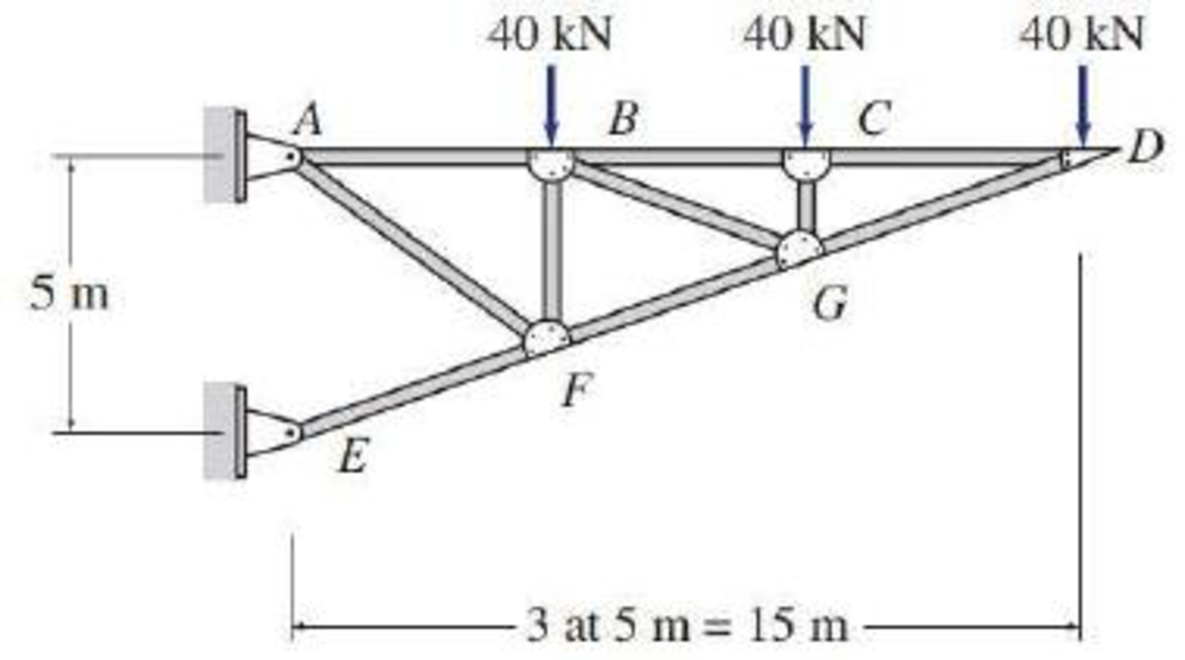# 4.6 through 4.28 Determine the force in each member of the truss shown by the method of joints. FIG. P4.17

#### Solutions

Chapter
Section
Chapter 4, Problem 17P
Textbook Problem
5 views

## 4.6 through 4.28 Determine the force in each member of the truss shown by the method of joints.FIG. P4.17To determine

Find the forces in the members of the truss by the method of joints.

### Explanation of Solution

Given information:

Apply the sign conventions for calculating reactions, forces and moments using the three equations of equilibrium as shown below.

• For summation of forces along x-direction is equal to zero (Fx=0), consider the forces acting towards right side as positive (+) and the forces acting towards left side as negative ().
• For summation of forces along y-direction is equal to zero (Fy=0), consider the upward force as positive (+) and the downward force as negative ().
• For summation of moment about a point is equal to zero (Matapoint=0), consider the clockwise moment as negative and the counter clockwise moment as positive.

Method of joints:

The negative value of force in any member indicates compression (C) and the positive value of force in any member indicates Tension (T).

Calculation:

Consider the forces in the member AB, BC, CD, DG, GF, and FE are FAB,FBC,FCD,FDG,FGF,FFE.

Show the free body diagram of the truss as shown in Figure 1.

Refer Figure 1.

Calculate the length of the member CG, BF using the relation:

DAAE=CDCGCG=CDDA×AECG=515×5CG=53

DAAE=BDBFBF=BDDA×AEBF=1015×5BF=103

Calculate the value of the angle CDG as follows:

θ=tan1(515)=18.43°

Calculate the value of the angle ABF as follows:

α=tan1[(103)5]=33.69°

Consider the joint D as shown in Figure 2.

Refer Figure 2.

Take the sum of the forces in the vertical direction as zero.

Fy=0FGDsinθ40=0FGDsin(18.43°)40=0FGD=40sin(18.43°)FGD=126.49kN(C)

Take the sum of the forces in the horizontal direction as zero.

Fx=0FGDcosθFCD=0FCD=FGDcos(18.43°)

Substitute 126.49kN for FGD.

FCD=(126.49)cos(18.43°)=120kN(T)

Consider the joint C as shown in Figure 3.

Refer Figure 3.

Take the sum of the forces in the vertical direction as zero.

Fy=0FCG40=0FCG=40FCG=40kN(C)

Take the sum of the forces in the horizontal direction as zero.

Fx=0FCB=FCD

Substitute 120kN for FCD.

FCB=120kN(T)

Show the joint G as shown in Figure 4.

Refer Figure 4.

Take the sum of the forces in the vertical direction as zero.

Fy=0FGC+FGBsin18.43°+FGDsin18.43°FGFsin18.43°=0(FGBFGF)sin18.43°=FGDsin18.43°FGCFGBFGF=FGDsin18.43°FGCsin18.43°FGBFGF=FGD3.163FGC

Substitute 40kN for GC and 126.49kN for FGD.

FGBFGF=(126.49)3.163×(40)FGBFGF=253        (1)

Take the sum of the forces in the horizontal direction as zero.

Fx=0FGBcos18.43°+FGDcos18

### Still sussing out bartleby?

Check out a sample textbook solution.

See a sample solution

#### The Solution to Your Study Problems

Bartleby provides explanations to thousands of textbook problems written by our experts, many with advanced degrees!

Get Started

Find more solutions based on key concepts
Explain what is meant by temperature and give examples of its important role in engineering analysis and design...

Engineering Fundamentals: An Introduction to Engineering (MindTap Course List)

Technology in Finance Technology enables individuals and businesses to conduct transactions in the finance indu...

Enhanced Discovering Computers 2017 (Shelly Cashman Series) (MindTap Course List)

What is an RFP, and how does it differ from an RFQ?

Systems Analysis and Design (Shelly Cashman Series) (MindTap Course List)

Explain the differences among data, information, and a database.

Database Systems: Design, Implementation, & Management

Determine the magnitude of the pin reaction at B. Neglect the weights of the members.

International Edition---engineering Mechanics: Statics, 4th Edition

What must be known to use the compound-rest taper turning method?

Precision Machining Technology (MindTap Course List)

If your motherboard supports ECC DDR3 memory, can you substitute non-ECC DDR3 memory?

A+ Guide to Hardware (Standalone Book) (MindTap Course List)